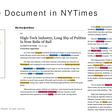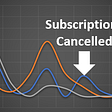# How to change the activation function in ANN model created using toolbox

I’ve created this model by editing the codes from the toolbox. The purpose of this model is to train the network with operating data from a turbine. the data is normalized and then the target will be set according to the actual fault occurrence which tagged as “1” and during normal operation “0”. I will be comparing the result of several training function, the number of neuron, the number of layers, and activation function.

`% This script assumes these variables are defined:%   data - input data.%   target - target data.% load dataload data.mat;load target.mat;x = data;t = target;% Choose a Training Function% For a list of all training functions type: help nntrain% 'trainlm' is usually fastest.% 'trainbr' takes longer but may be better for challenging problems.% 'trainscg' uses less memory. NFTOOL falls back to this in low memory situations.trainFcn = 'trainbr';  % Bayesian Regularization% Create a Feedforward NetworkhiddenLayerSize = 18;net = feedforwardnet (hiddenLayerSize,trainFcn);% Setup Division of Data for Training, Validation, TestingRandStream.setGlobalStream(RandStream('mt19937ar','seed',1)); % to get constant resultnet.divideFcn = 'divideblock'; % Divide targets into three sets using blocks of indicesnet.divideParam.trainRatio = 70/100;net.divideParam.valRatio = 15/100;net.divideParam.testRatio = 15/100;%TRAINING PARAMETERSnet.trainParam.show=50;  %# of ephocs in displaynet.trainParam.lr=0.05;  %learning ratenet.trainParam.epochs=10000;  %max epochsnet.trainParam.goal=0.05^2;  %training goalnet.performFcn='mse';  %Name of a network performance function %type help nnperformance% Train the Network[net,tr] = train(net,x,t); % Test the Networky = net(x);e = gsubtract(t,y);performance = perform(net,t,y)% View the Networkview(net)`

The questions are: Is it correct to use this code below and will it affect the function of my model?

`RandStream.setGlobalStream(RandStream('mt19937ar','seed',1)); % to get constant result`

How to add another hidden layer? How to change the activation function for each layer? What is the best plot to show the capability of the ANN model to detect the fault of the turbine earlier than existing control system. Please advise me if there are anything that can be corrected/improved.

Matlabsolutions.com provide latest MatLab Homework Help,MatLab Assignment Help for students, engineers and researchers in Multiple Branches like ECE, EEE, CSE, Mechanical, Civil with 100% output.Matlab Code for B.E, B.Tech,M.E,M.Tech, Ph.D. Scholars with 100% privacy guaranteed. Get MATLAB projects with source code for your learning and research.

You are approaching the problem in exactly the wrong way.

The multilayer perceptron with one hidden layer is a universal approximator. The only reason to use more than one hidden layer is to reduce the total number of unknown weights by reducing the total number of hidden nodes (i.e., H1+H2 < H).

Ntrn training pairs of I-dimensional inputs and O-dimensional output targets yields Ntrneq = Ntrn*O training equations. The best way to obtain a robust design that tends to be resistant to noise, interference, measurement and transcription error is to MINIMIZE the number of unknown weights that yield an acceptable solution. If possible, Nw << Ntrneq is desirable

`1. Use FITNET (calls FEEDFORWARDNET) for regression and curve-fitting 2. Use PATTERNNET (calls FEEDFORWARDNET) for classification and pattern-recognition 3. You have a classification problem. Start with the simple code in     help patternnet     doc patternnet 4. If there are c classes, the target matrix columns should be columns of eye(c): O = c. 5. The relationship between trueclass indices 1:c and the target columns is target           = ind2vec(trueclassindices); trueclassindices = vec2ind(target);`

--

--

--

## More from Technical Source

Simple! That is me, a simple person. I am passionate about knowledge and reading. That’s why I have decided to write and share a bit of my life and thoughts to.

Love podcasts or audiobooks? Learn on the go with our new app.

## MACHINE LEARNING (1100101)b: LET’S LEARN ABOUT LEARNING## AWS DeepAR algorithm## How Qchain Will Use Natural Language Processing## K Means Algorithm explained with an example## DO 2D GANS KNOW 3D SHAPE? UNSUPERVISED 3D SHAPE RECONSTRUCTION FROM 2D IMAGE GANS## Using PyTorch for building a Convolutional Neural Network (CNN) model## How to measure customer love and keep revenue rolling## Understanding Deep Self-attention Mechanism in Convolution Neural Networks## Technical Source

Simple! That is me, a simple person. I am passionate about knowledge and reading. That’s why I have decided to write and share a bit of my life and thoughts to.

## Fix Attribute Error and Runtime Error in tensorflow and keras | Beginner Must Read